Courses

# Test: Motion in a Straight Line- 1

## 30 Questions MCQ Test Physics Class 11 | Test: Motion in a Straight Line- 1

Description
This mock test of Test: Motion in a Straight Line- 1 for JEE helps you for every JEE entrance exam. This contains 30 Multiple Choice Questions for JEE Test: Motion in a Straight Line- 1 (mcq) to study with solutions a complete question bank. The solved questions answers in this Test: Motion in a Straight Line- 1 quiz give you a good mix of easy questions and tough questions. JEE students definitely take this Test: Motion in a Straight Line- 1 exercise for a better result in the exam. You can find other Test: Motion in a Straight Line- 1 extra questions, long questions & short questions for JEE on EduRev as well by searching above.
QUESTION: 1

### The position of an object moving in a straight line can be specified with reference to

Solution:

Explanation:To describe motion along a straight line, we can choose an axis, say X-axis, so that it coincides with the path of the object. We then measure the position of the object with reference to a conveniently chosen origin, say O, Positions to the right of O are taken as positive and to the left of O, as negative.

QUESTION: 2

### If the position- time graph is a straight line parallel to the time axis

Solution:

Explanation:Position-time graph of horizontal straight line parallel to time axis represents that the position of the body does not changes with the passage of time. So it represents the rest state of motion.It means velocity of object is zero.

QUESTION: 3

### A truck is coming towards you from a distance of 1000m on a straight road at time t=0. At time t=10 seconds it is at a distance of 900 m. The average velocity in m/sec is

Solution: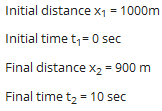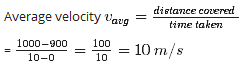QUESTION: 4

A truck has a velocity of 3 m /s at time t=0. It accelerates at 3 m / s2 on seeing police .What is its velocity in m/s at a time of 2 sec

Solution: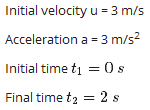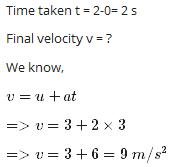QUESTION: 5

A truck covers 40.0 m in 8.50 s while smoothly slowing down to a final speed of 2.80 m/s. Find its original speed in m/s

Solution: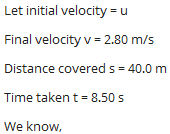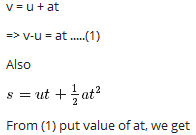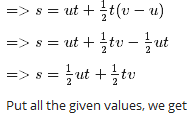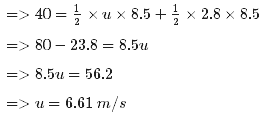QUESTION: 6

For motion in a straight line

Solution:

Explanation:To describe motion along a straight line, we can choose an axis, say X-axis, so that it coincides with the path of the object. We then measure the position of the object with reference to a conveniently chosen origin, say O,  Positions to the right of O are taken as positive and to the left of O, as negative.

QUESTION: 7

The average acceleration a over a time interval is defined as

Solution:

Explanation:

Acceleration is the rate of change of velocity with time.It is a vector quantity.

Average acceleration is the change in velocity per unit time over an interval of time.

Let initial velocity at time interval t​​​​​​1 is v​​​​​​1 and final velocity at time interval t​​​​​2 is v​​​​​​2 Then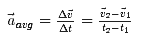QUESTION: 8

A truck is coming towards you from a distance of 1000m on a straight road, at time t=0. It reaches you in 100sec. The average speed in m/s is

Solution: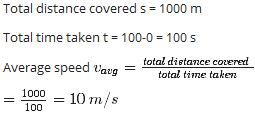QUESTION: 9

A stone thrown from the top of a building is given an initial velocity of 20.0 m/s straight upward. Determine the time in seconds at which the stone reaches its maximum height.

g =9.8 m / sec2

Solution: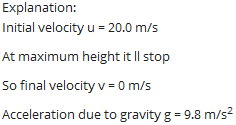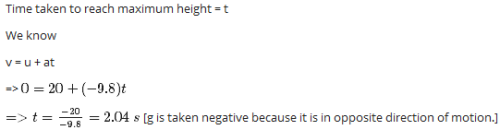QUESTION: 10

A truck covers 40.0 m in 8.50 s while smoothly slowing down to a final speed of 2.80 m/s. Find its acceleration in m/s2

Solution: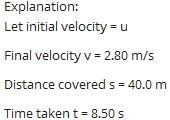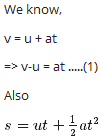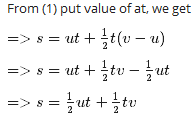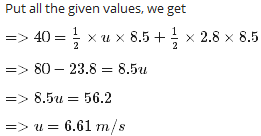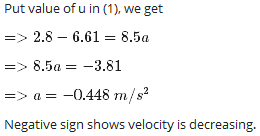QUESTION: 11

Path length is defined as.

Solution:

Explanation:Path length is defined as the total length of the path traversed by an object.

Unlike displacement, which is the total distance an object travels from a starting point, path length is the total distance travelled, regardless of where it travelled.

QUESTION: 12

For motion with uniform acceleration, v-t graph is

Solution:

Explanation:When velocity – time graph is plotted for an object moving with uniform acceleration, the slope of the graph is a straight line.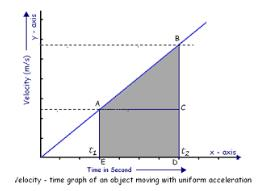QUESTION: 13

A truck is coming towards you from a distance of 1000m on a straight road, at time t=0. The driver stops for a second near a poster. He reaches you in 100 sec. The average speed in m/s is

Solution: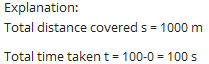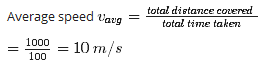QUESTION: 14

A stone thrown from the top of a building is given an initial velocity of 20.0 m/s straight upward. Determine the maximum height it travels in meters. g =9.8 m / sec2

Solution:

Explanation:

Initial velocity u = 20.0 m/s

At maximum height stone ll  be stopped,

So final velocity v = 0 m/s

Acceleration due to gravity a = g =

-9.8 m/s​​​​​​2 (-ve Because it is in opposite direction of motion)

Let maximum height = s

We know,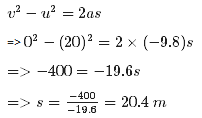QUESTION: 15

A body moving with uniform acceleration has a velocity of 12.0 cm/s in the positive x direction when its x coordinate is 3.00 cm. If its x coordinate 2.00 s later is -5.00 cm, what is the magnitude of its acceleration in cm/s2 ?

Solution: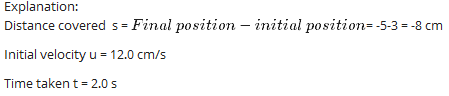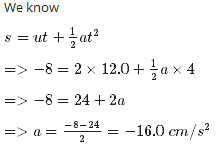QUESTION: 16

Path length is a

Solution:

Explanation:Path length has no particular direction and it depends upon the path chosen to reach the destination where displacement of the destination is absolute no matter what path is used to get there. So it is scaler.

QUESTION: 17

The area under the velocity-time curve between times t1 and t2 is equal to the.

Solution:

Explanation:The area under the velocity-time curve between times t1​​​and t​​​​​​2 is equal to the displacement of the object during that interval of time.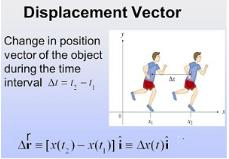QUESTION: 18

With reference to the figure which shows a particle moving along a straight line , y axis represents the position and x axis represents time, if x1 = 18m, The average velocity at if x1 in m/s is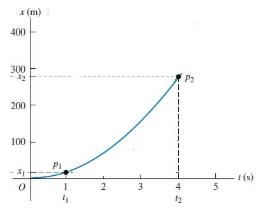Solution:

Explanation:

Average velocity can be defined as the displacement divided by the time.

Total displacement d = 18 m

Time taken t = 1 s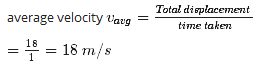QUESTION: 19

A stone thrown from the top of a building is given an initial velocity of 20.0 m/s straight upward. Determine the time in seconds at which the stone returns to the height from which it was thrown.

g = 9.8 m / sec2

Solution: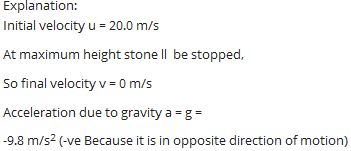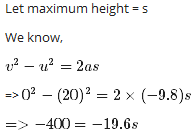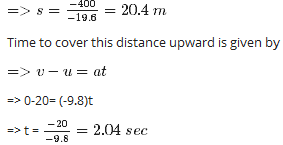Again to reach same level from where it has been thrown it have to cover same distance downward.

For this initial velocity u = 0

s = 20.4 m

a = 9.8 m/s​​​​​​2 (+be because motion is in same direction).

So,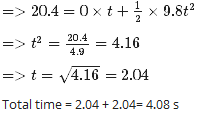QUESTION: 20

In Kinematics we study

Solution:

Explanation:Kinematics is a branch of classical mechanics that describes the motion of points, bodies (objects), and systems of bodies (groups of objects) without considering the mass of each or the forces that caused the motion.

QUESTION: 21

In which of the following examples of motion, can the body be considered approximately a point object?

Solution:

Explanation:Point object is an expression used in kinematics branch of physics: it is an object whose dimensions are ignored or neglected while considering its motion.The size of a carriage is very small as compared to the distance between two stations. Therefore, the carriage can be treated as a point sized object.

QUESTION: 22

The dimensional formula for acceleration is

Solution:
QUESTION: 23

For motion in 3 dimensions we need

Solution:

Explanation:Motion is a change in position of an object with time. In order to specify position, we need to use a reference point and a set of axes. It is convenient to choose a rectangular coordinate system consisting of three mutually perpenducular axes, labelled X-, Y-, and Z- axes.

The point of intersection of these three axes is called origin (O) and serves as the reference point. The coordinates (x, y. z) of an object describe the position of the object with respect to this coordinate system.

To measure time, we position a clock in this system. This coordinate system along with a clock constitutes a frame of reference.

QUESTION: 24

A drag racer starts her car from rest and accelerates at 10.0 m/s2 for the entire distance of 400 m .How long did it take the race car to travel this distance in s?

Solution:

Explanation:

Initial velocity u = 0

Acceleration a = 10.0 m/s​​​​​​2

Distance covered s = 400 m

Time taken t = ?

We know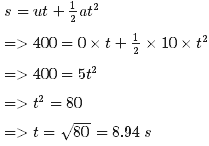QUESTION: 25

A stone thrown from the top of a building is given an initial velocity of 20.0 m/s straight upward. Determine the time in seconds at which the stone reaches its maximum height.

g =9.8 m / sec2

Solution:

Explanation:

Initial velocity u = 20.0 m/s

At maximum height it ll stop

So final velocity v = 0 m/s

Acceleration due to gravity g = 9.8 m/s​​​​​​2

Time taken to reach maximum height = t

We know

v = u + at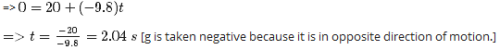QUESTION: 26

Displacement is a

Solution:

Explanation:Displacement vector is a vector which gives the position of a point with reference to a point other than the origin of the coordinate system.

QUESTION: 27

Name the instrument used to measure instantaneous speed of a vehicle.

Solution:

Explanation:A speedometer or a speed meter is a gauge that measures and displays the instantaneous speed of a vehicle. Now universally fitted to motor vehicles, they started to be available as options in the 1900s, and as standard equipment from about 1910 onwards.

Speedometers for other vehicles have specific names and use other means of sensing speed. For a boat, this is a pit log. For an aircraft, this is an airspeed indicator.

Charles Babbage is credited with creating an early type of a speedometer, which were usually fitted to locomotives.

QUESTION: 28

Motion along a straight line is called __________.

Solution:

Explanation:Rectilinear motion is another name for straight-line motion. This type of motion describes the movement of a particle or a body. A body is said to experience rectilinear motion if any two particles of the body travel the same distance along two parallel straight lines.

QUESTION: 29

An object is said to be in uniform motion in a straight line if its displacement

Solution:

Explanation:Uniform motion is the kind of motion in which a body covers equal displacement in equal intervals of time. It does not matter how small the time intervals are, as long as the displacements covered are equal.

If a body is involved in rectilinear motion and the motion is uniform, then the acceleration of the body must be zero.

QUESTION: 30

A truck has a velocity of 2 m /s at time t=0. It accelerates at 2 m / s2 on seeing police .What is its velocity in m/s at a time of 2 sec

Solution:

Explanation:

Initial velocity u = 2 m/s

final velocity = v m/s

Time duration = final time - initial time = 2-0 = 2 s

acceleration a = 2 m/s​​​​​​2

We know,

v = u + at

=> v = 2+2x2

=> v = 6 m/s